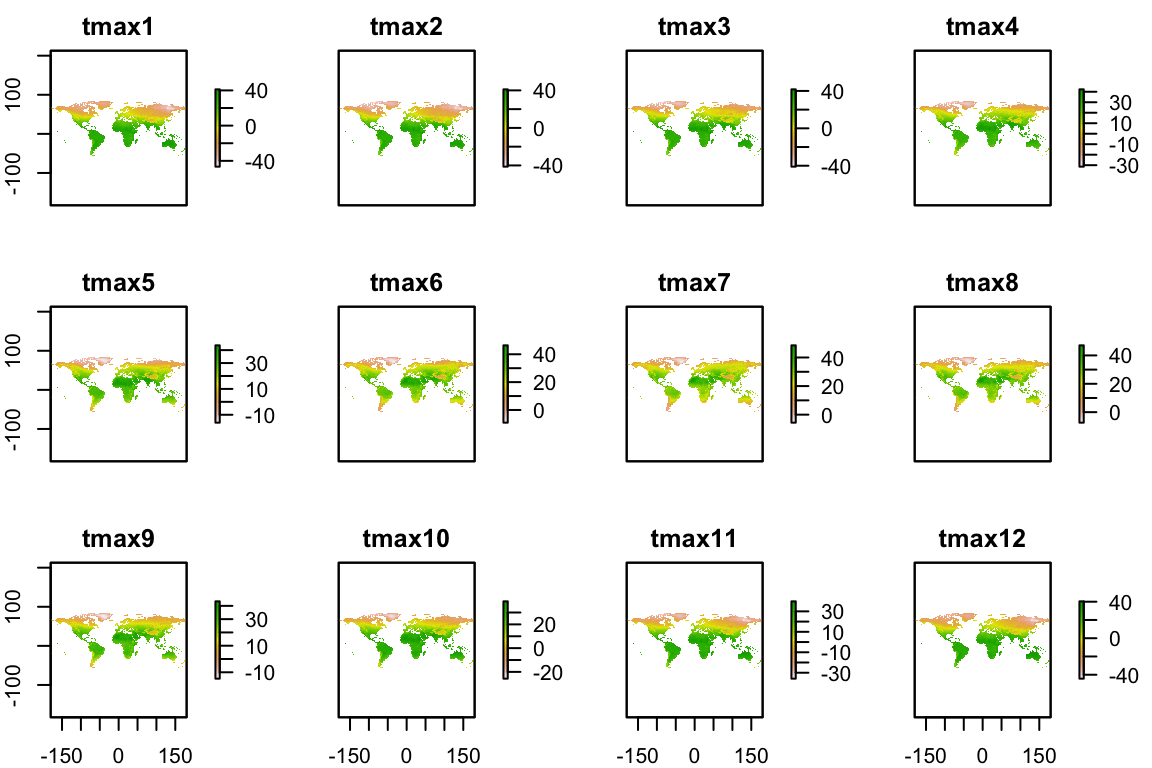# Objective

In this case study we used the temperature data from WorldCLim source (which are near-global rasters of various climate variables) to identify the hottest country on each continent, excluding Antarctica.

# Data and Method

### Libraries

Include required packages (or install if necessary):

``````library(raster)
library(sf)
library(sp)
library(spData)
library(tidyverse)
library(dplyr)``````

### Prepare the Climate Data

get the climate data (world) from the spData package

``data(world)``

filter out Antarctica and convert to an sp data format

``````filtered_world = filter(world, continent != "Antarctica")
sp_data =  as(filtered_world,"Spatial")``````

load the WorldClim maximum temperature dataset at the lowest resolution

``tmax_monthly <-getData(name = "worldclim", var="tmax", res=10)``

inspect tmax_monthly by plotting it. Note: the units are not in Celsius, so they must be converted: \(C = unit*0.10\)

``````gain(tmax_monthly) <- 0.1
plot(tmax_monthly)``````temperature map per month over the course of a year

Find the annual maximum temperature in each pixel of the raster stack, and change the column tmax_annual to the name tmax (for convenience)

``````tmax_annual=max(tmax_monthly)
names(tmax_annual) <- "tmax"``````

### Calculate the Maximum Temperature Observed in Each Country

Find the maximum temperature observed in each country as a spatial polygon object

``````max_temp_country = raster::extract(x=tmax_annual,y=world,fun=max,na.rm=T,small=T,sp=T)
max_temp_country = st_as_sf(max_temp_country)``````

# Result

### Plot the results

Using ggplot we can create plot the maximum temperature in each country

``````plot <- ggplot(max_temp_country,aes(fill=tmax))+
geom_sf() +
ggtitle("Annual Maximum Temperature for Each Country") +
scale_fill_viridis_c(name="Annual\nMaximum\nTemperature (C)") +
xlab('Latitude') +
ylab('Longitude') +
theme(legend.position ='bottom',plot.title=element_text(size=8,color="black"))``````